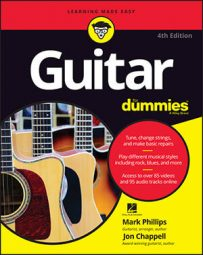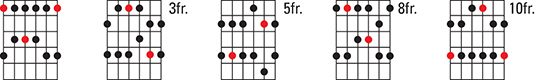##### Guitar For DummiesThe pentatonic minor scale is the principal scale used in rock and blues guitar soloing. As its name implies, it consists of five notes (unlike common major and minor scales, which consist of seven notes). On the fretboard, the finger pattern of the pentatonic scale resembles the shape of a box, so pentatonic minor scales on the guitar sometimes are referred to as boxes, or box positions.

If you use fretted notes only (no open strings), scale patterns are movable. The lowest all-fretted pentatonic minor scale found on the fretboard is F pentatonic minor (starting from the 1st fret of the low E string), as shown in the first box. The red dots show the F notes — the note the scale is named for.

The naming note of a scale can be called the first degree of the scale or the tonic note. The black dots show the scale’s other degrees. The degrees of the pentatonic minor scale are generally referred to by their intervallic distance above the tonic of a normal, seven-note major scale, as follows: the first degree is the “1” or the tonic; the next degree is the ♭3 (a note a half step, or one fret, lower than the 3rd degree of a major scale); the next degree is the 4th; the next the 5th; and the last the ♭7. Any pentatonic minor scale thus has the formula 1-♭3-4-5-♭7; hence, the F pentatonic minor scale is F-A flat-B flat-C-E flat.The second box above uses the same five notes as the first box, but it starts from the ♭3 (A flat) instead of the 1. The third box in the figure uses the same notes, but it begins at the 4 (B flat). The fourth box in the figure starts from the 5 (C), and the last box starts from the ♭7 (E flat). Refer to the fret number alongside the boxes to make sure you start each scale from the correct fret. These finger patterns consist of two notes per string, which are generally played with the 1st and 3rd left-hand fingers or the 1st and 4th (depending on whether the notes are two or three frets apart).

When playing a rock or blues solo in the key of F, use any or all of these five boxes. Interestingly, even if the song is in the key of F major, you solo using the F pentatonic minor scale; this produces a bluesy effect. Because all the notes in the boxes are fretted, the scales are movable. So if, for example, you need to play in the key of G, simply move the patterns up two frets (because G is two frets higher than F).